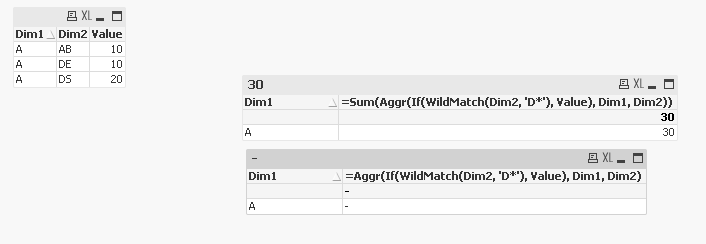QlikView Scripting

Discussion Board for collaboration on QlikView Scripting.

Announcements
QlikWorld 2020: Join us May 11 - 14, 2020 in Phoenix, AZ. Register early and save \$400. Learn More
Contributor

Which AGGR to use?

I recently had a query regarding how to use the AGGR function.  Although I managed to resolve, I still don't understand it.

Can anyone explain in very simple terms the difference between the following

Aggr(Sum ......)

&

Sum(Aggr(Sum .......)

I cannot seem to get my head around them.

When & Why do you use them?

Examples welcome to demonstrate.

Cheers

Phil

Tags (2)
2 RepliesMVP & Luminary

Re: Which AGGR to use?

I'm not sure that I could explain it very well and therefore I suggest to look on the following postings:

AGGR...

Calculated Dimensions

In short: Aggr(Sum ......) will be used within calculated dimensions and Sum(Aggr(Sum .......) within expressions.

- MarcusMVP

Re: Which AGGR to use?

When you are using Aggr(Sum(....), the assumption is that there is only one value available after the inner Sum() is completed and that one value will be displayed. If for any reasons, there are more than one values, you will see - (null). Aggr(Sum(...) can be written like this Only(Aggr(Sum(....)) as they essentially mean the same exact thing.

Now Sum(Aggr(Sum(...)) is saying that if you have multiple values, then the expression should Sum those values and display it.

Here is an example for you to look atI wanted to sum those rows where Dim2 began with D.... Since there are two such rows, the one without Sum() gave me null. Where as the one with Sum() gave me the right sum.

Does this make sense?